# Getting started with colorhcplot

#### February 19, 2018

The colorhcplot package is a convenient tool for plotting colorful dendrograms where clusters, or sample groups, are highlighted by different colors. In order to generate a colorful dendrogram, colorhcplot() function requires 2 mandatory arguments: hc and fac:

• hc is the result of a hclust() call

• fac is a factor defining the grouping

The number of leaves of the dendrogram has to be identical to the length of fac (i.e., length(hc$labels) == length(fac) has to be TRUE). Also, the optional colors argument (if supplied) has to have a length of 1 (single color) or equal to the length of the levels of fac. ## Install install.packages("colorhcplot") library(colorhcplot) ## Example 1: using the USArrests dataset The first example is based on the USArrests dataset and compares the results of the standard plot method applied to a hclust-class object and the output of colorhcplot(). The use of simple arguments is illustrated. data(USArrests) hc <- hclust(dist(USArrests), "ave") fac <- as.factor(c(rep("group 1", 10), rep("group 2", 10), rep("unknown", 30))) plot(hc)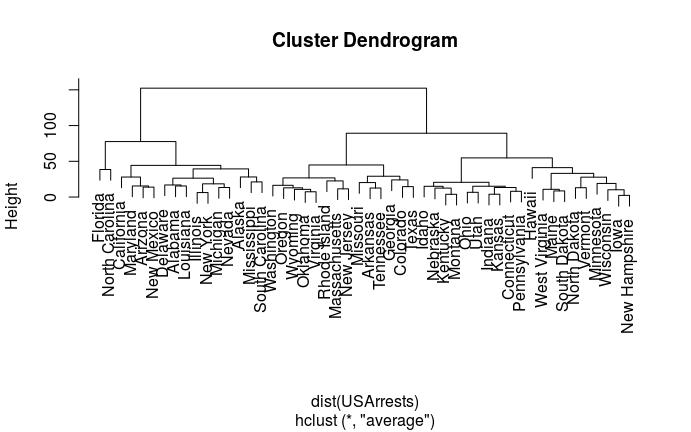colorhcplot(hc, fac)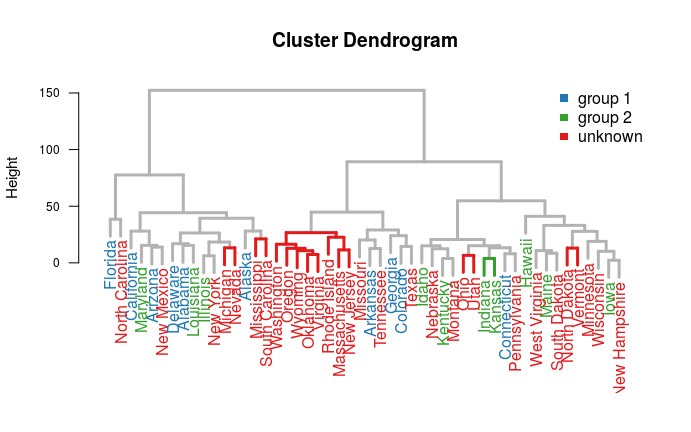colorhcplot(hc, fac, hang = -1, lab.cex = 0.8)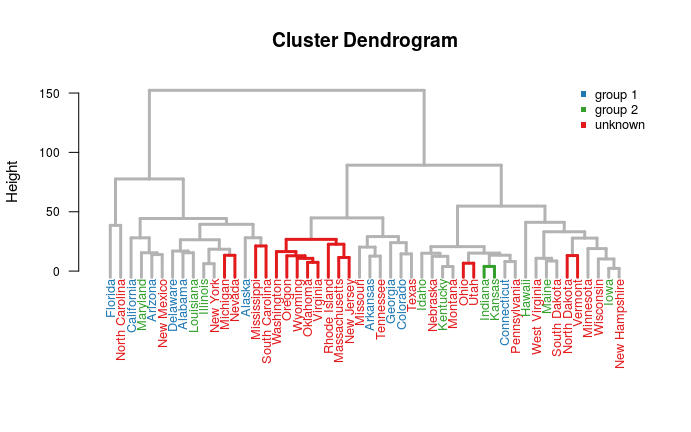### Example 2: use the “ward.D2” algorithm and the UScitiesD dataset The second example is based on the UScitiesD dataset. Here we show how to specify custom colors for the colorhcplot() call, using the colors argument. data(UScitiesD) hcity.D2 <- hclust(UScitiesD, "ward.D2") fac.D2 <-as.factor(c(rep("group1", 3), rep("group2", 7))) plot(hcity.D2, hang=-1)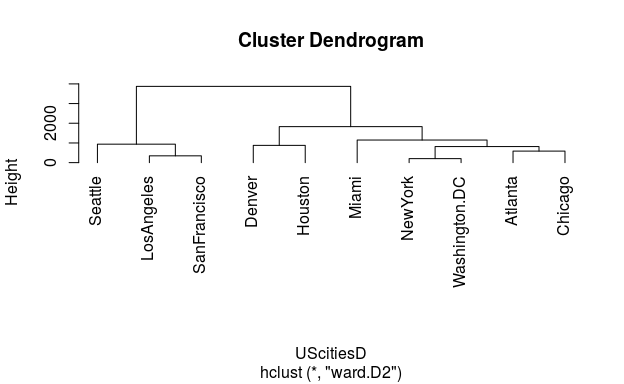colorhcplot(hcity.D2, fac.D2, color = c("chartreuse2", "orange2"))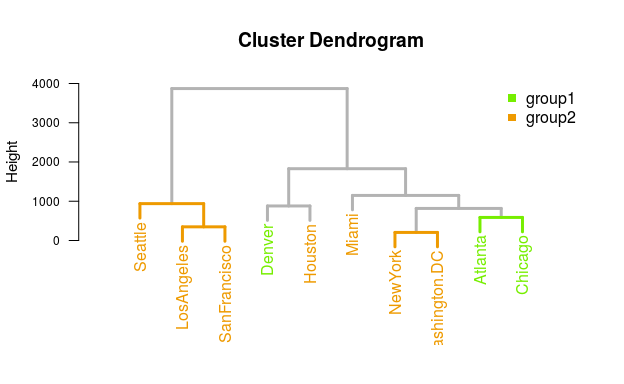colorhcplot(hcity.D2, fac.D2, color = "gray30", lab.cex = 1.2, lab.mar = 0.75)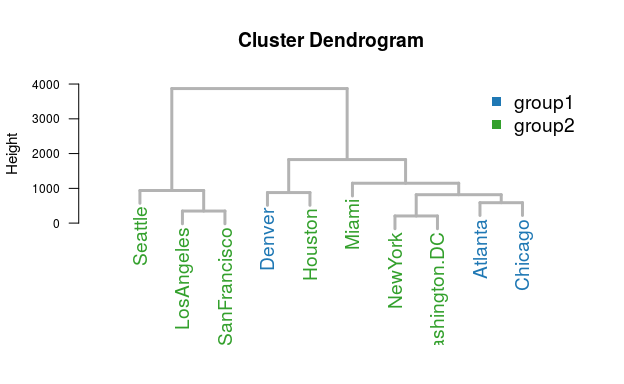## Example 3: use gene expression data The third example is based on a sample gene expression dataset, which is included in the colorhcplot package. This illustrate how to use colorhcplot() for exploration and analysis of genomic data. data(geneData, package="colorhcplot") exprs <- geneData$exprs
fac <- geneData\$fac
hc <- hclust(dist(t(exprs)))
colorhcplot(hc, fac, main ="default", col = "gray10")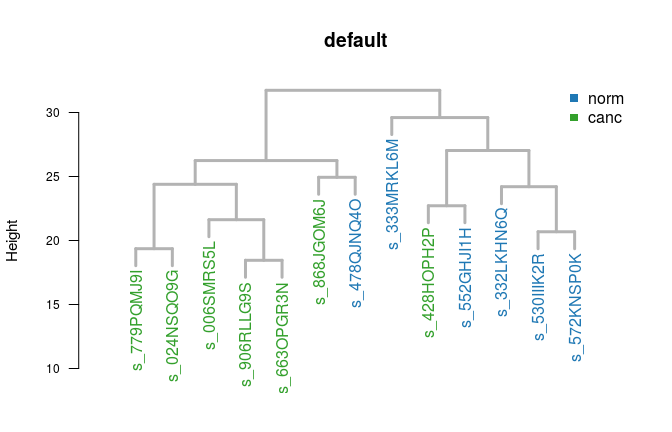colorhcplot(hc, fac, main="Control vs. Tumor Samples")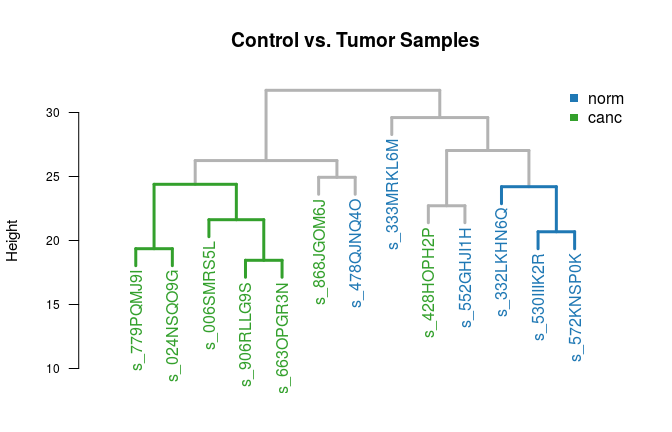## SessionInfo

sessionInfo()
## R version 3.4.3 (2017-11-30)
## Platform: x86_64-pc-linux-gnu (64-bit)
## Running under: Ubuntu 16.04.3 LTS
##
## Matrix products: default
## BLAS: /usr/lib/libblas/libblas.so.3.6.0
## LAPACK: /usr/lib/lapack/liblapack.so.3.6.0
##
## locale:
##   LC_CTYPE=en_US.UTF-8       LC_NUMERIC=C
##   LC_TIME=en_US.UTF-8        LC_COLLATE=C
##   LC_MONETARY=en_US.UTF-8    LC_MESSAGES=en_US.UTF-8
##   LC_PAPER=en_US.UTF-8       LC_NAME=C
##  digest_0.6.14   evaluate_0.10.1